Скачать презентацию 8 -11 Surface Area of Pyramids and Cones

f5928b61250491e508da895e59dfd5f9.ppt

• Количество слайдов: 20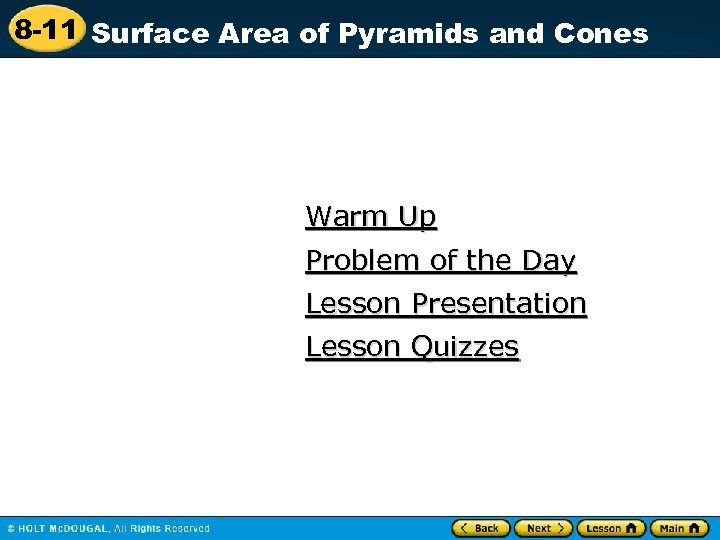8 -11 Surface Area of Pyramids and Cones Warm Up Problem of the Day Lesson Presentation Lesson Quizzes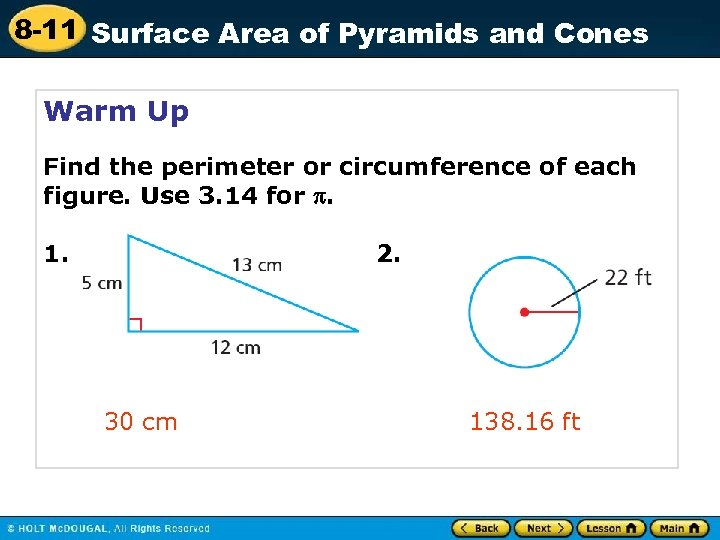8 -11 Surface Area of Pyramids and Cones Warm Up Find the perimeter or circumference of each figure. Use 3. 14 for . 1. 2. 30 cm 138. 16 ft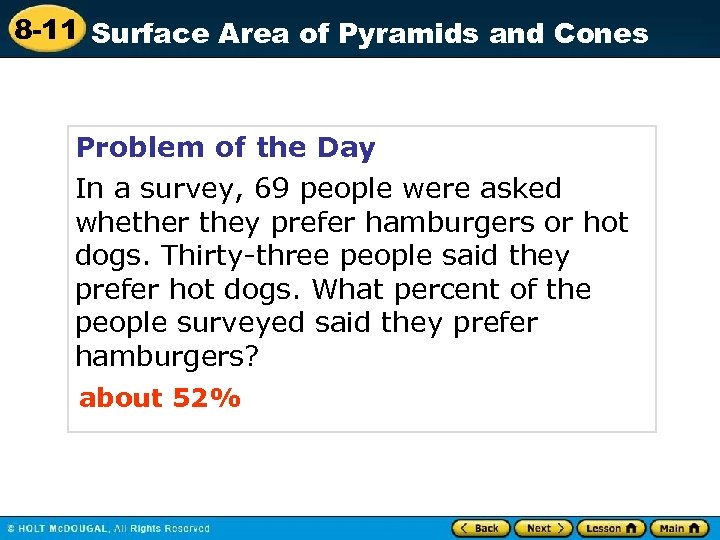8 -11 Surface Area of Pyramids and Cones Problem of the Day In a survey, 69 people were asked whether they prefer hamburgers or hot dogs. Thirty-three people said they prefer hot dogs. What percent of the people surveyed said they prefer hamburgers? about 52%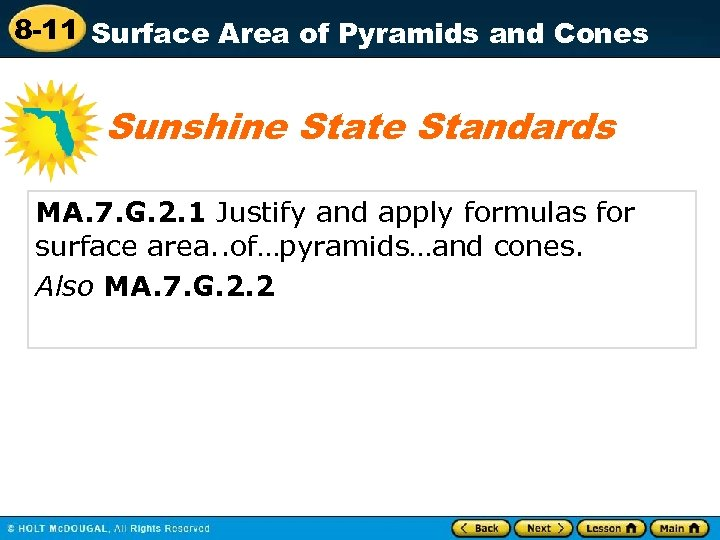8 -11 Surface Area of Pyramids and Cones Sunshine State Standards MA. 7. G. 2. 1 Justify and apply formulas for surface area. . of…pyramids…and cones. Also MA. 7. G. 2. 2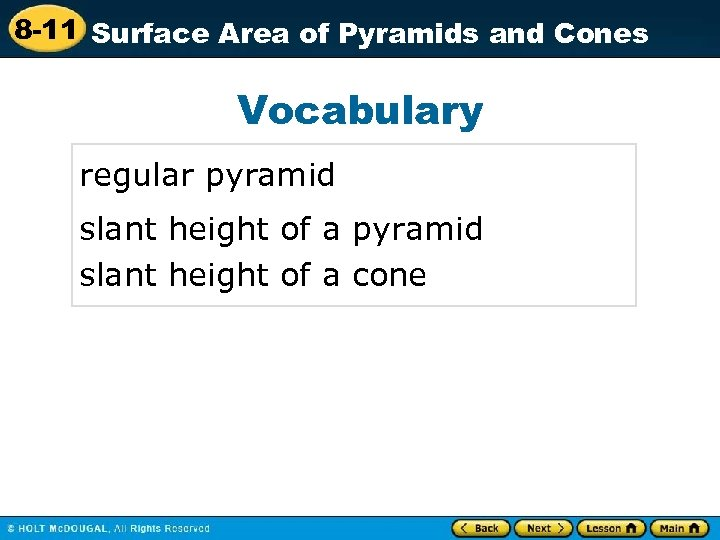8 -11 Surface Area of Pyramids and Cones Vocabulary regular pyramid slant height of a cone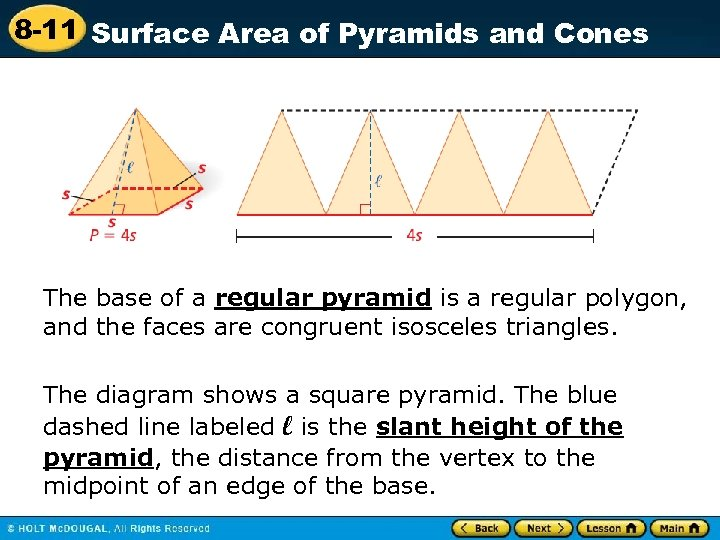8 -11 Surface Area of Pyramids and Cones The base of a regular pyramid is a regular polygon, and the faces are congruent isosceles triangles. The diagram shows a square pyramid. The blue dashed line labeled l is the slant height of the pyramid, the distance from the vertex to the midpoint of an edge of the base.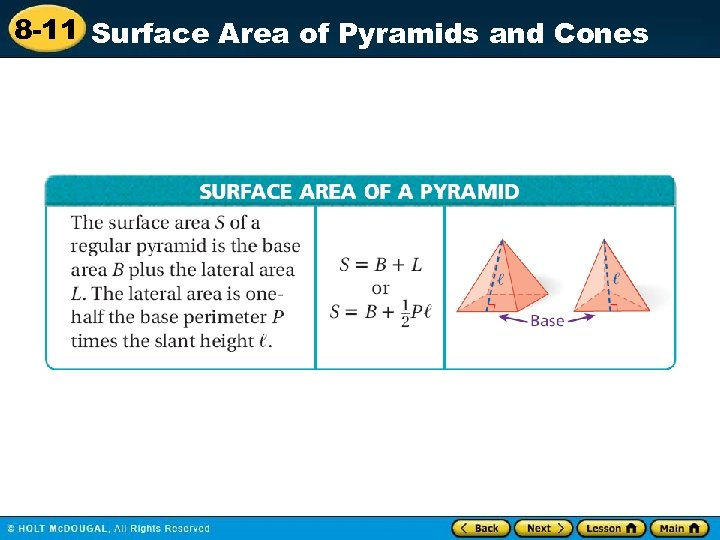8 -11 Surface Area of Pyramids and Cones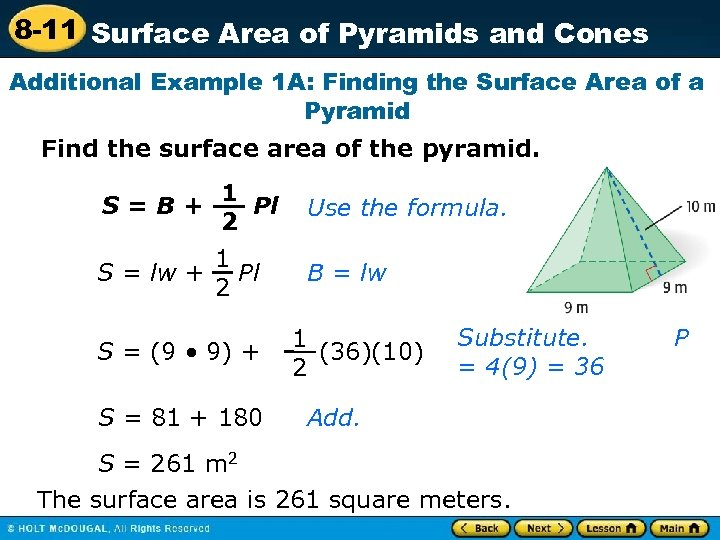8 -11 Surface Area of Pyramids and Cones Additional Example 1 A: Finding the Surface Area of a Pyramid Find the surface area of the pyramid. S=B+ 1 Pl 2 1 S = lw + Pl 2 S = (9 • 9) + S = 81 + 180 Use the formula. B = lw 1 (36)(10) 2 Substitute. = 4(9) = 36 Add. S = 261 m 2 The surface area is 261 square meters. P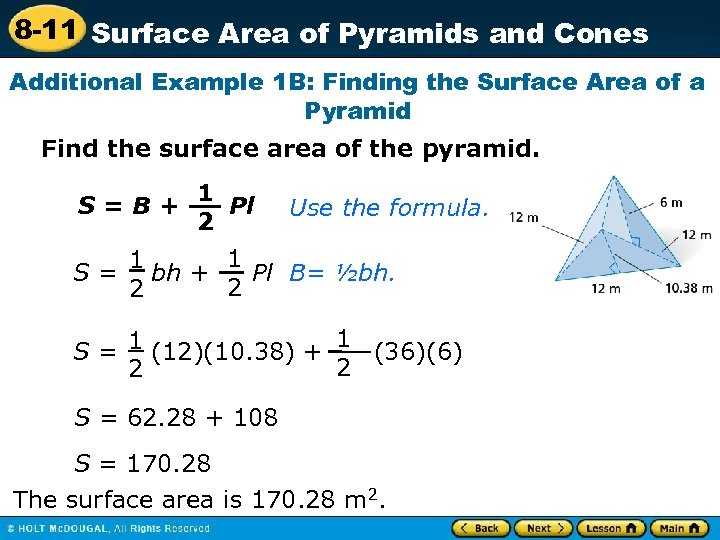8 -11 Surface Area of Pyramids and Cones Additional Example 1 B: Finding the Surface Area of a Pyramid Find the surface area of the pyramid. S=B+ 1 Pl 2 Use the formula. 1 1 S = bh + Pl B= ½bh. 2 2 1 (12)(10. 38) + 1 (36)(6) S= 2 2 S = 62. 28 + 108 S = 170. 28 The surface area is 170. 28 m 2.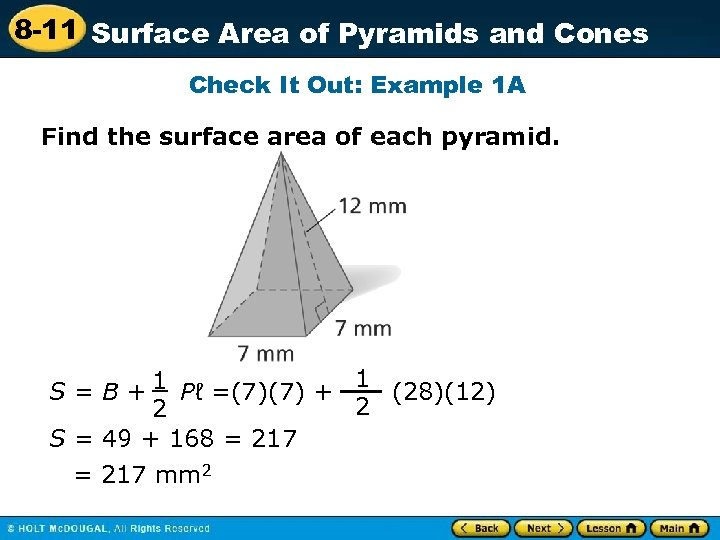8 -11 Surface Area of Pyramids and Cones Check It Out: Example 1 A Find the surface area of each pyramid. 1 S = B + 1 Pℓ =(7)(7) + (28)(12) 2 2 S = 49 + 168 = 217 mm 2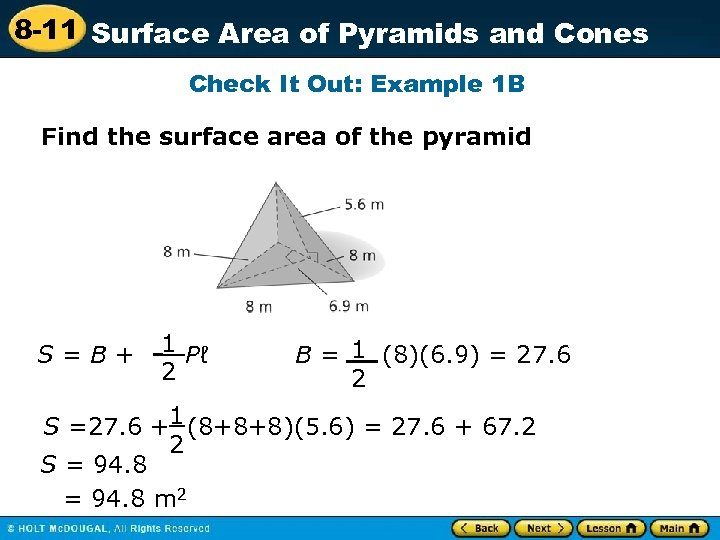8 -11 Surface Area of Pyramids and Cones Check It Out: Example 1 B Find the surface area of the pyramid S=B+ 1 Pℓ 2 B = 1 (8)(6. 9) = 27. 6 2 S =27. 6 +1 (8+8+8)(5. 6) = 27. 6 + 67. 2 2 S = 94. 8 m 2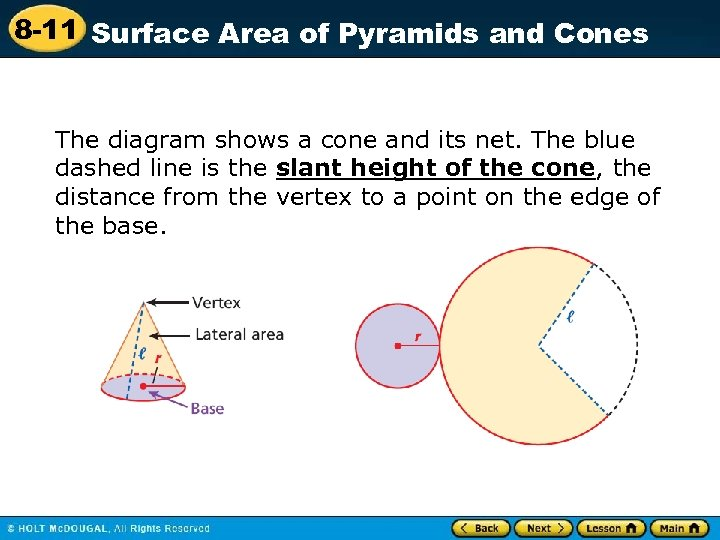8 -11 Surface Area of Pyramids and Cones The diagram shows a cone and its net. The blue dashed line is the slant height of the cone, the distance from the vertex to a point on the edge of the base.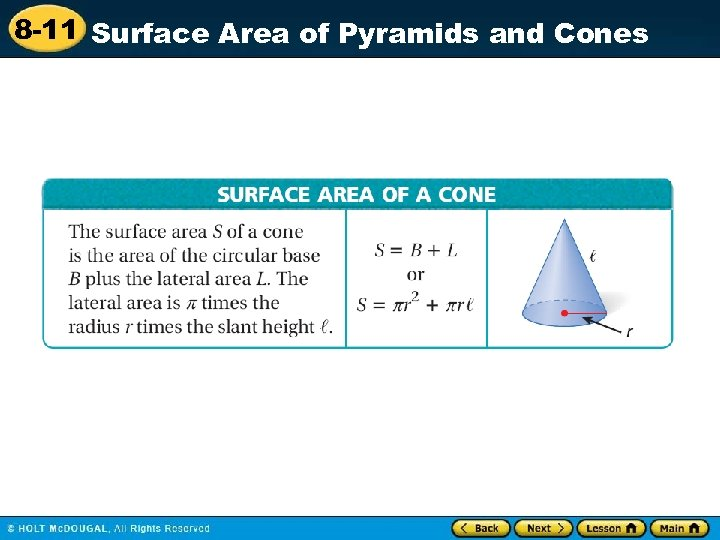8 -11 Surface Area of Pyramids and Cones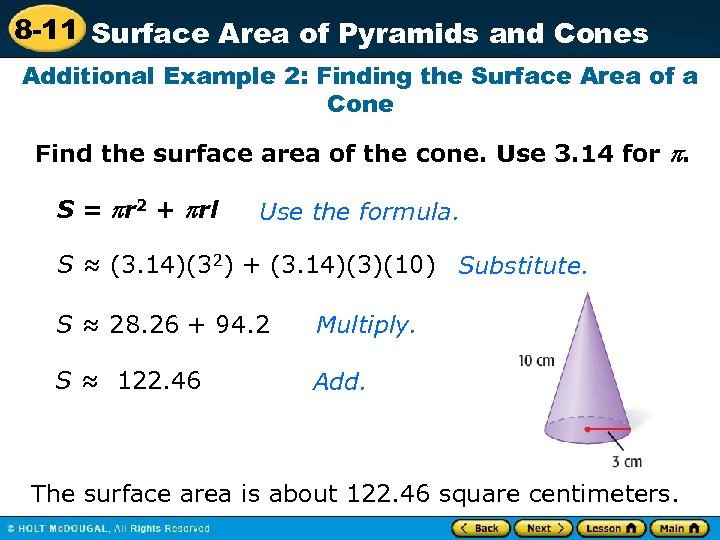8 -11 Surface Area of Pyramids and Cones Additional Example 2: Finding the Surface Area of a Cone Find the surface area of the cone. Use 3. 14 for . S = r 2 + rl Use the formula. S ≈ (3. 14)(32) + (3. 14)(3)(10) Substitute. S ≈ 28. 26 + 94. 2 Multiply. S ≈ 122. 46 Add. The surface area is about 122. 46 square centimeters.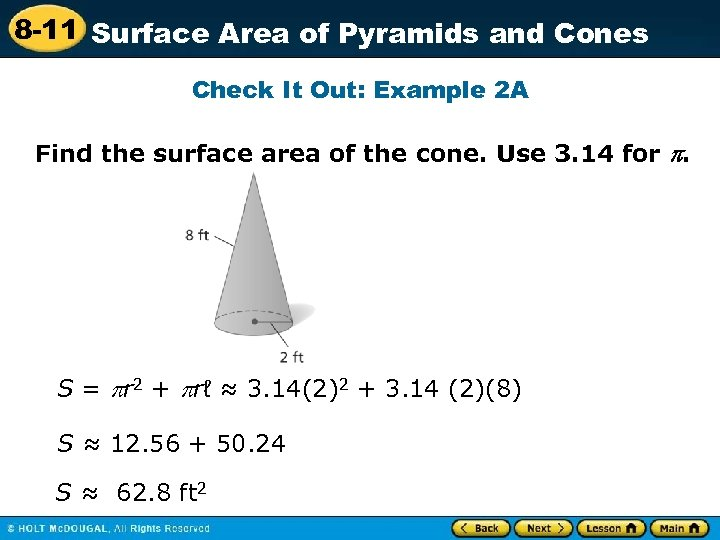8 -11 Surface Area of Pyramids and Cones Check It Out: Example 2 A Find the surface area of the cone. Use 3. 14 for . S = r 2 + rℓ ≈ 3. 14(2)2 + 3. 14 (2)(8) S ≈ 12. 56 + 50. 24 S ≈ 62. 8 ft 2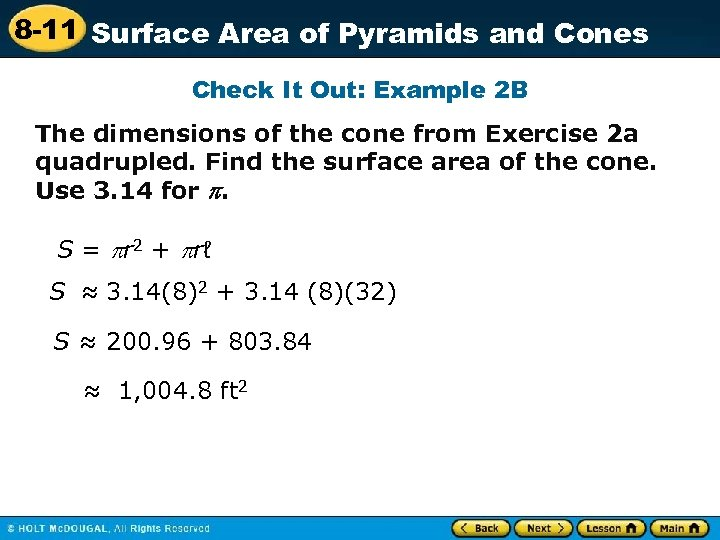8 -11 Surface Area of Pyramids and Cones Check It Out: Example 2 B The dimensions of the cone from Exercise 2 a quadrupled. Find the surface area of the cone. Use 3. 14 for . S = r 2 + rℓ S ≈ 3. 14(8)2 + 3. 14 (8)(32) S ≈ 200. 96 + 803. 84 ≈ 1, 004. 8 ft 2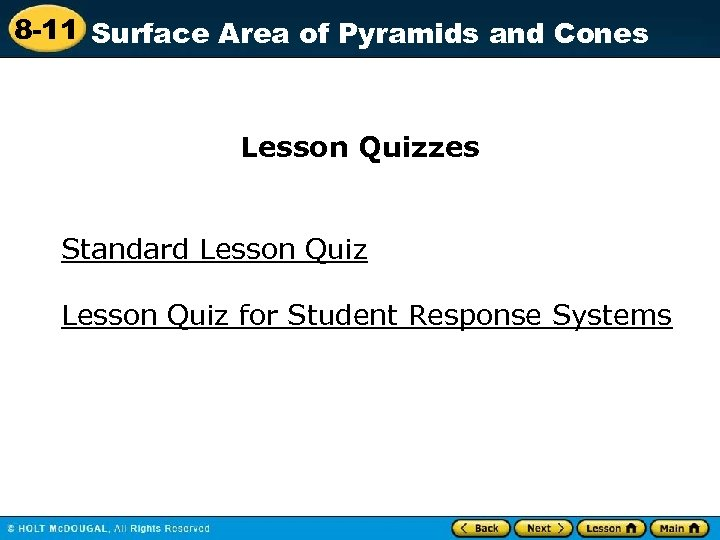8 -11 Surface Area of Pyramids and Cones Lesson Quizzes Standard Lesson Quiz for Student Response Systems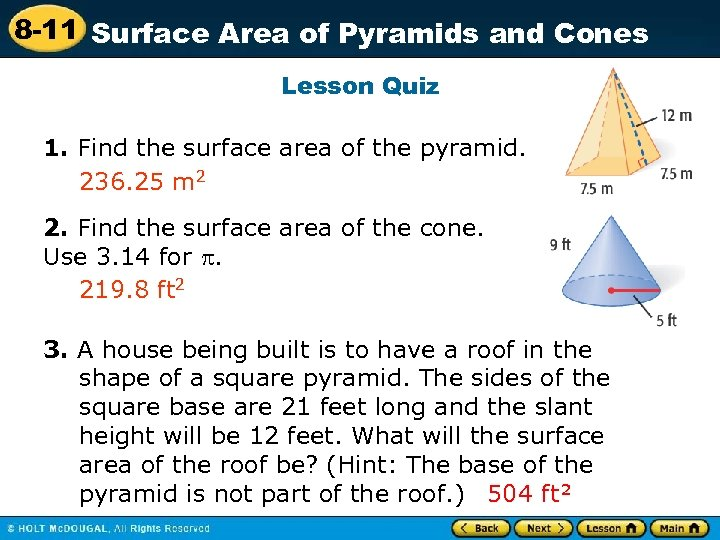8 -11 Surface Area of Pyramids and Cones Lesson Quiz 1. Find the surface area of the pyramid. 236. 25 m 2 2. Find the surface area of the cone. Use 3. 14 for . 219. 8 ft 2 3. A house being built is to have a roof in the shape of a square pyramid. The sides of the square base are 21 feet long and the slant height will be 12 feet. What will the surface area of the roof be? (Hint: The base of the pyramid is not part of the roof. ) 504 ft²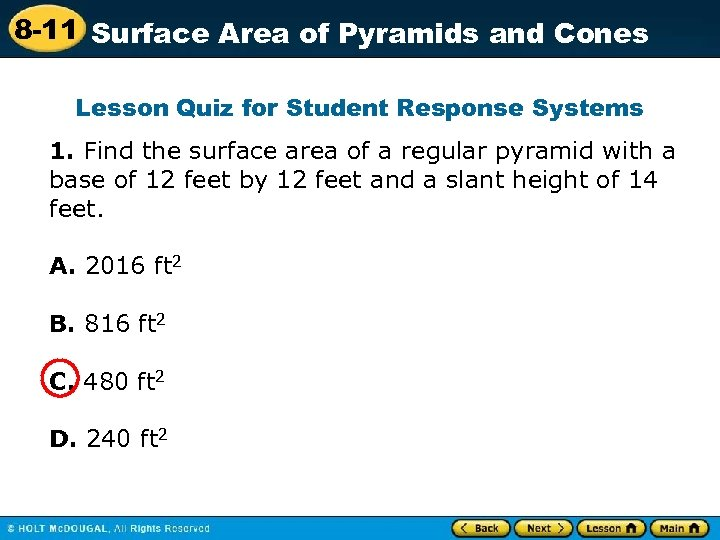8 -11 Surface Area of Pyramids and Cones Lesson Quiz for Student Response Systems 1. Find the surface area of a regular pyramid with a base of 12 feet by 12 feet and a slant height of 14 feet. A. 2016 ft 2 B. 816 ft 2 C. 480 ft 2 D. 240 ft 2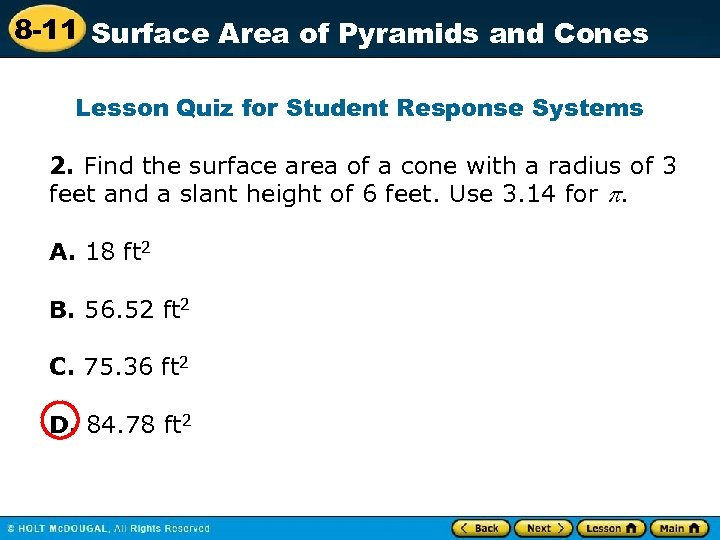8 -11 Surface Area of Pyramids and Cones Lesson Quiz for Student Response Systems 2. Find the surface area of a cone with a radius of 3 feet and a slant height of 6 feet. Use 3. 14 for . A. 18 ft 2 B. 56. 52 ft 2 C. 75. 36 ft 2 D. 84. 78 ft 2